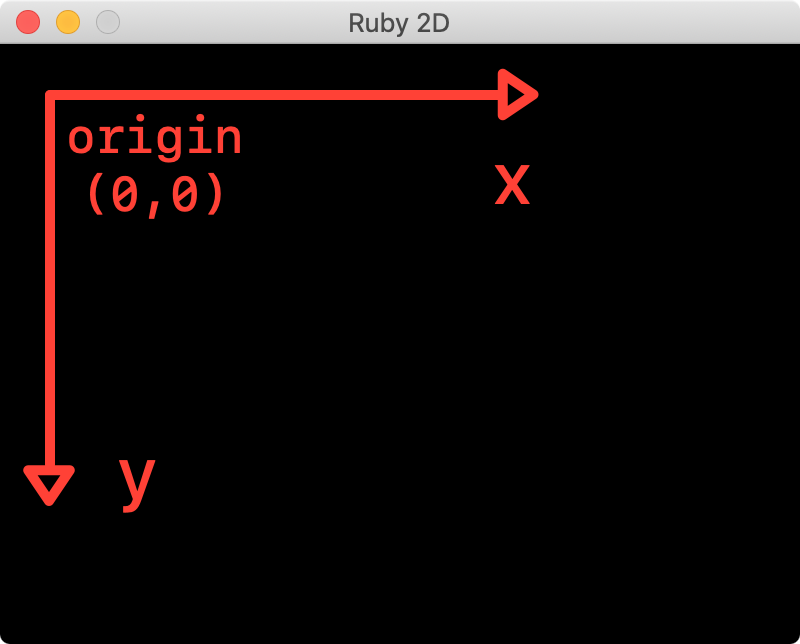# 2D basics

Learn the fundamentals of 2D graphics

Before we start making some graphics, let’s cover the basics.

# Coordinate system

First, it’s important to know that visible objects drawn in the window follow a coordinate system. Instead of something you might be familiar with from your math classes, this system has its origin, or `(0, 0)`, in the upper-left corner, and the x and y values get larger toward the bottom-right. This might seem odd, but it’s actually common in 2D computer graphics.In this documentation, `x` is the x-coordinate and `y` is the y-coordinate. When an object has multiple pairs of x and y coordinates, they will be numbered, for example: `x1`, `y1` or `x2`, `y2`.

All objects that can be drawn in the window are added automatically. When you called `Triangle.new` in the “get started” tutorial, its `add` method was called for you, which added the triangle to the window. If you want to remove an object from the window, simply call `remove`. Here’s an example showing how to remove, then add a shape back to the window:

``````s = Square.new  # a square is created and added to the window
s.remove  # square is removed...
``````

All objects can be removed from the window by simply calling `clear`:

``````Triangle.new
Square.new
clear  # everything now gone from the window
``````

# Depth

Objects are drawn in the order they are created. For example, if you call `Triangle.new` and then `Square.new`, the triangle will be behind the square. You can override this behavior by setting a “z-index” for the object. An object with a higher index will be “above” (in front) of another with a lower value. All objects start with a z-index of `0`.

For example, to make the following triangle appear above the square, we can set it’s z-index like so:

``````t = Triangle.new
s = Square.new
t.z = 1
``````

# Contains

You can check if a point is within the bounds of an object by calling its `contains?` method like so:

``````s = Square.new(x: 50, y: 50, size: 100)
s.contains? 75, 75  # returns true
s.contains? 10, 20  # returns false
``````

# Example of moving a square shape with keys

``````require 'ruby2d'

# Define a square shape.
@square = Square.new(x: 10, y: 20, size: 25, color: 'blue')

# Define the initial speed (and direction).
@x_speed = 0
@y_speed = 0

# Define what happens when a specific key is pressed.
# Each keypress influences on the  movement along the x and y axis.
on :key_down do |event|
if event.key == 'j'
@x_speed = -2
@y_speed = 0
elsif event.key == 'l'
@x_speed = 2
@y_speed = 0
elsif event.key == 'i'
@x_speed = 0
@y_speed = -2
elsif event.key == 'k'
@x_speed = 0
@y_speed = 2
end
end

update do
@square.x += @x_speed
@square.y += @y_speed
end

show
``````

Continue to the next topic ▸